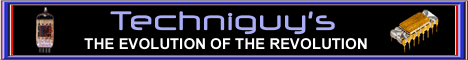## UNDERSTANDING BITS, BYTES, Ks, & MEGS

#### The units of measure for computers

Since electronic logic circuits can only see an "on" or "off" condition, not number values, all computers use "binary" as their basic language. Binary has only two digits..... 0 and 1. This can be represented in logic circuits as "on" and "off" or plus and minus. The smallest binary unit in a computer is call a "bit" and can be either a 0 or a 1 depending on the magnetic polarity of its memory location.

In the decimal system that we all use, each digit position has a value. Moving from the decimal point on the right to the left we have units position with a value of 1 x the digit. Next to that we have the tens position with a value of 10 x the digit, and next is the hundreds position with a value of 100 x the digit. Binary is similar but since we can only have a 0 or a 1 as the digit, it works like this...... position 1 = 1. position 2 = 2. If we have a 1 in both of those positions, then that's 1 + 2 = 3 so we don't need a 3s position. The value of the third position is 4. by having different combinations of bits on or off, and by observing the value of the positions, we can now display any digit from 0 to 7 using only 0s and 1s. By adding another position which is the 8s position, we can now count from 0 to 15 using only 0s and 1s. Binary (0101) would equal 5 because we have the 1s and the 4s position turned on. (1010) would equal 10 because we have the 8s and the 2s position turned on. Just to clear up any confusion here the positions are like this; (8s 4s 2s 1s). If all positions are on like this (1111) this is 8+4+2+1 = 15 and when you add the 0 (0000) you have 16 numeric values known as a "halfbyte". This system is expanded by using two halfbytes together to create up to 256 values which is enough to represent all the the characters both upper and lower case, numbers, and special characters contained in any keyboard. This in binary; (1111)(1111) is two halfbytes and known as a "byte" and is equal to the Hex value of 255. "HEXadecimal" is the basic "machine" language of all computers and each keyboard character is assigned a hex number. A byte is always made up of 2 hex digits to give a value of 0 to 255. You may have seen hex numbers being used in RGB color codes and wondered what those letters from a to f were doing in there. The decimal system has digits from 0 to 9 but the HEX system has digits from 0 to F.

I won't go into hex to decimal conversions here as I think it might get too confusing, other than to say that the decimal system is based on powers of 10, where the hex system is based on powers of 16. The hex system uses digits of 0 to 9, then a, b, c, d, e, f before you get to 10 so "F" in hex is equal to 15 decimal and 10 in hex is equal to 16 in decimal. Hex instead of decimal is used in computers because of the easy way it converts to binary, the only system that computers can work with.

You now understand what a "byte" is, or you are completely confused so lets go on with the easy part....lol.

If you have a 1K (Kilobyte) memory in your computer, then you can store 1000 bytes of information (or 1000 characters from your keyboard). You're most likely going to need more than that if you intend to do anything more that add 2 and 2.

If you have a 1Meg memory in your computer then you can store 1 million bytes of information (or 1 million characters from your keyboard). Now we have something we can use but if we're building a website, we are not going to be able to make too many pages for it with only a million bytes of storage space. Even the Webtv pagebuilder gives you 3 million bytes or 3 Megabytes or "Megs" of storage space.

What if you need more than 999 Megs? Then you're going to need a "Gigabyte" or 1,000 Megs, or 1,000,000 K, or 1,000,000,000 bytes. (that's a billion bytes)

I hope that clears up the question of "bits", "bytes", "Ks", and "Megs". Now lets get busy and build those 10 Gigabyte websites...lol. Any questions?

You may now dismiss yourself with your back button, or click the banner at the top to go to my main page.